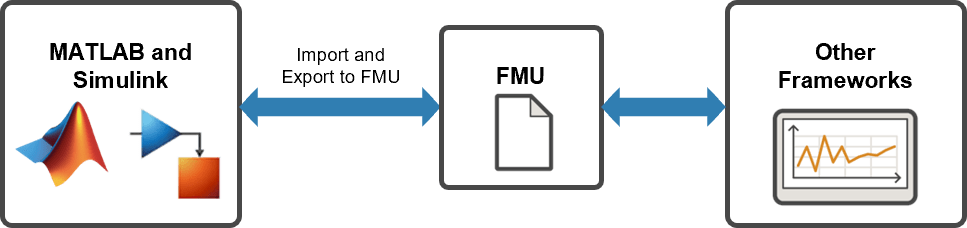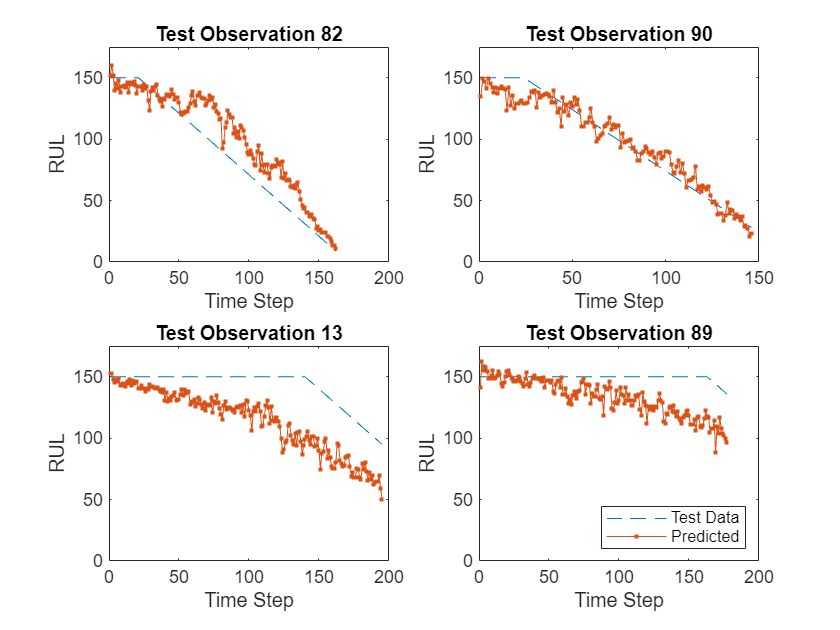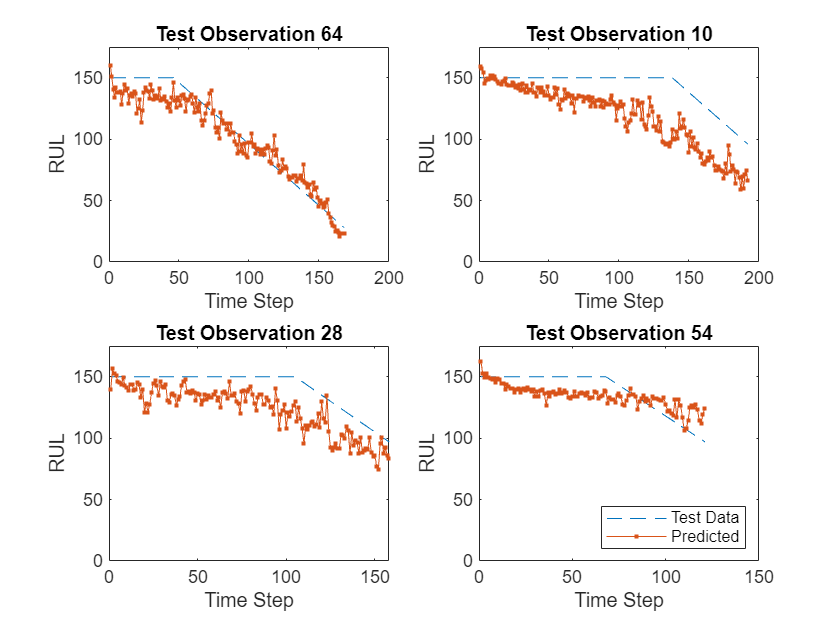# Export Network to FMU

Since R2023b

This example shows how to export a trained network as a Functional Mock-up Unit (FMU).

An FMU is a file that contains a simulation model that adheres to the Functional Mock-up Interface (FMI) standard . Software that implements the FMI standard can use FMU files for model exchange or co-simulation. MATLAB® and Simulink® support exporting trained deep neural networks as FMUs. This example shows how to take a network trained using Deep Learning Toolbox™, implemented and tested using Simulink, and export it to FMU for use with other simulation software.This example uses a pretrained LSTM network to predict the remaining useful life (RUL) of an engine, measured in cycles. The LSTM network consists of an LSTM layer with 200 hidden units, followed by a fully connected layer of size 50 and a dropout layer with dropout probability 0.5.The network was trained using the Turbofan Engine Degradation Simulation Data Set as described in . The training data contains simulated time series data for 100 engines. Each sequence varies in length and corresponds to a full run to failure (RTF) instance. The test data contains 100 partial sequences and corresponding values of the remaining useful life at the end of each sequence. For more information on training the network, see the example Sequence-to-Sequence Regression Using Deep Learning.

`net = coder.loadDeepLearningNetwork("rulNetwork.mat");`

This section summarizes the steps to download and prepare the test data that this example uses. For more information on the Turbofan Engine Degradation Simulation data set and the preprocessing steps, see Sequence-to-Sequence Regression Using Deep Learning.

Create a directory to store the Turbofan Engine Degradation Simulation data set.

```dataFolder = fullfile(tempdir,"turbofan"); if ~exist(dataFolder,"dir") mkdir(dataFolder); end```

```filename = matlab.internal.examples.downloadSupportFile("nnet","data/TurbofanEngineDegradationSimulationData.zip"); unzip(filename,dataFolder)```

Preprocess the data using the `processTurboFanData` function provided at the end of this example. The `processTurboFanData` function returns:

• structure `simin` containing the data values and an empty time vector

• cell array `YValidate` containing target sequences

• array `sequenceLengths` containing the lengths of the sequences in `YValidate`

`[simin,YValidate,sequenceLengths] = processTurboFanData(dataFolder);`

The Simulink model for predicting the remaining useful life of a turbofan engine is shown. The model uses the Predict block from the Deep Neural Networks library that imports the trained network from the `rulNetwork` MAT-file. Additionally, the `Mini-batch size` parameter of the block is set to `1`.

```model = "rulPredict"; open_system(model)```

### Run the Simulation

`sim(model);`

The output `YPred` of the Simulink model contains the predicted remaining useful life values from the network. This output is first trimmed to remove the results from the zero-padded values and then converted to a cell array.

```maxSequenceLen = max(sequenceLengths); YPred_cell = squeeze(mat2cell(YPred,1,maxSequenceLen,ones(1,100))); for t = 1:numel(sequenceLengths) YPred_cell{t}(:,sequenceLengths(t) + 1:end) = []; end```

Plot the predicted RUL values for four randomly-selected observations and compare them to the validation data. The supporting function `rulExamplePlots` is provided at the end of this example.

```observationIdx = randperm(100,4); rulExamplePlots(observationIdx,YValidate,YPred_cell);```### Prepare Model for Export

To be exported as an FMU, a deep learning model in Simulink must meet the following requirements.

• The network used by the Predict block must support code generation without using any third-party libraries.

• The input and output signals, parameters, and their elements of the model must be `double`, `int32`, `boolean`, or `string`.

• The simulation target language must be C.

• The solver type must be `fixed-step`.

Check that the network supports library-free code generation.

`analyzeNetworkForCodegen(net,TargetLibrary="none")`
``` Supported _________ none "Yes" ```

For a list of the networks, layers, and classes supported for code generation, see Networks and Layers Supported for Code Generation (MATLAB Coder).

The network used by the Predict block outputs single-precision numeric values. As `single` is not an allowed output data type when exporting to FMU, add a Data Type Conversion block to convert the output to `double`.

```delete_line(model,"Predict/1","Outport/1") add_block("simulink/Commonly Used Blocks/Data Type Conversion",model+"/CastToDouble") set_param(model+"/CastToDouble",OutDataTypeStr="double") add_line(model,"Predict/1","CastToDouble/1") add_line(model,"CastToDouble/1","Outport/1")```

The simulation target language defines the language of the generated code. Check that the target language is C. If the target language is not C, set it using `set_param(activeConfigObj,``"SimTargetLang"``,``"C++"``)`.

`get_param(model,"SimTargetLang")`
```ans = 'C' ```

By default, Simulink automatically selects a variable-step solver. Set the solver type to `fixed-step`.

`set_param(model,SolverType="fixed-step")`

Replace the From Workspace input block and replace with an Inport block. Set Inport port dimensions to match the dimensions of the data in `simin` and realign the layout of the model.

```replace_block(model,"FromWorkspace","Inport","noprompt") set_param(model+"/From Workspace",Name="In1",PortDimensions="[17 303]") Simulink.BlockDiagram.arrangeSystem(model)```

### Export Model to FMU

Using the `exportToFMU2CS` function, export the model to an FMU. The `exportToFMU2CS` (Simulink Compiler) function creates an .fmu file in the current folder with the same name as the model.

`exportToFMU2CS("rulPredict");`
```Setting System Target to FMU Co-Simulation for model 'rulPredict'. Setting Hardware Implementation > Device Type to 'MATLAB Host' for model 'rulPredict'. ### 'GenerateReport' is disabled for Co-Simulation Standalone FMU Export. ### 'GenerateComments' is disabled for Co-Simulation Standalone FMU Export. Build Summary Top model targets built: Model Action Rebuild Reason ============================================================================================ rulPredict Code generated and compiled. Code generation information file does not exist. 1 of 1 models built (0 models already up to date) Build duration: 0h 1m 57.954s ### Model was successfully exported to co-simulation standalone FMU: 'C:\TEMP\rulPredict.fmu'. ```

Close the model without saving.

`bdclose(model)`

### Test FMU (Optional)

To ensure that the FMU operates correctly, you can reimport it into Simulink.

Open the original model.

`open_system(model)`

Replace the Predict block with an FMU block and set the FMU name parameter to the name of the FMU file.

```replace_block(model,"Predict","FMU","noprompt") set_param(model+"/Predict",Name="FMU",FMUName="rulPredict.fmu")```

Connect the blocks and remove unused lines.

```add_line(model,"From Workspace/1","FMU/1") delete_line(find_system(model,FindAll="on",Type="line",Connected="off"))```

As co-simulating with an FMU in Simulink introduces a time delay of one time step, increase the `StopTime` of the model by one time step. In this example, the `StopTime` is increased from `9.9` to `10` as the solver sample time is `0.1`. For more information on this delay, see Why there is a time delay of one time step while co-simulating a FMU in Simulink?.

`set_param(model,StopTime="10");`

Run the simulation.

`sim(model);`

Remove the first element of the predicted values due to the time delay introduced by the FMU block.

`YPred = YPred(:,:,2:end);`

Plot the predicted RUL values for four randomly-selected observations and compare them to the validation data.

```YPred_cell = squeeze(mat2cell(YPred,1,maxSequenceLen,ones(1,100))); for t = 1:length(sequenceLengths) YPred_cell{t}(:,sequenceLengths(t) + 1:end) = []; end observationIdx = randperm(100,4); rulExamplePlots(observationIdx,YValidate,YPred_cell);```### Supporting Functions

#### Process TurboFan Data

The `processTurboFanData` function reads the turbofan training and testing data and returns structure `simin` containing normalized validation data and an empty time vector, cell array `YValidate` containing target sequences, and array `sequenceLengths` containing the lengths of the sequences in `YValidate`. To make the input validation data compatible with Simulink code generation, the sequence lengths for each of the independent 100 observations are zero-padded to create uniformly-sized, 17-by-303 input arrays. The padded values are then converted to a 17-by-303-by-100 numeric array. To import this data into the Simulink model, specify a structure variable containing the data values and an empty time vector. During simulation, the input for the first time step is read from the first 17-by-303 element of the array. The value for the second time step is read from the second element, and so on, for a total of 100 steps.

The `processTurboFanData` function uses the functions `processTurboFanDataTrain` and `processTurboFanDataTest`, which are attached to this example as supporting files. Open the example as a live script to use these functions.

```function [simin,YValidate,sequenceLengths] = processTurboFanData(dataFolder) % Determine the mean and variance of the training data. filenamePredictors = fullfile(dataFolder,"train_FD001.txt"); [XTrain] = processTurboFanDataTrain(filenamePredictors); m = min([XTrain{:}],[],2); M = max([XTrain{:}],[],2); idxConstant = M == m; for i = 1:numel(XTrain) XTrain{i}(idxConstant,:) = []; end mu = mean([XTrain{:}],2); sig = std([XTrain{:}],0,2); % Read the validation data and normalize. filenamePredictors = fullfile(dataFolder,"test_FD001.txt"); filenameResponses = fullfile(dataFolder,"RUL_FD001.txt"); [XValidate,YValidate] = processTurboFanDataTest(filenamePredictors,filenameResponses); thr = 150; for i = 1:numel(XValidate) XValidate{i}(idxConstant,:) = []; XValidate{i} = (XValidate{i} - mu) ./ sig; YValidate{i}(YValidate{i} > thr) = thr; end % Pad the sequences. sequenceLengths = cellfun(@length,XValidate,UniformOutput=true); maxSequenceLen = max(sequenceLengths); padFcn = @(x) [x,zeros(size(x,1),maxSequenceLen-size(x,2))]; XValidatePad = cellfun(padFcn,XValidate,UniformOutput=false); % Store the validation data and an emtpy time vector in a structure. simin.time = []; simin.signals.values = cell2mat(reshape(XValidatePad,1,1,[])); simin.signals.dimensions = size(XValidatePad{1}); end```

#### Plot Remaining Useful Life

The `rulExamplePlots` function plots RUL values, comparing target values against predictions.

```function rulExamplePlots(observationIdx,YTest,YPred) N = numel(observationIdx); figure for i = 1:N subplot(N/2,2,i) plot(YTest{observationIdx(i)},'--') hold on plot(YPred{observationIdx(i)},'.-') hold off ylim([0 175]) title("Test Observation " + observationIdx(i)) xlabel("Time Step") ylabel("RUL") end legend(["Test Data" "Predicted"],Location="southeast") end```

### References

1. The Functional Mock-up Interface (FMI) Standard Specification, Version 3.0, https://fmi-standard.org/.

2. Saxena, Abhinav, Kai Goebel, Don Simon, and Neil Eklund. "Damage propagation modeling for aircraft engine run-to-failure simulation." In Prognostics and Health Management, 2008. PHM 2008. International Conference on, pp. 1-9. IEEE, 2008.